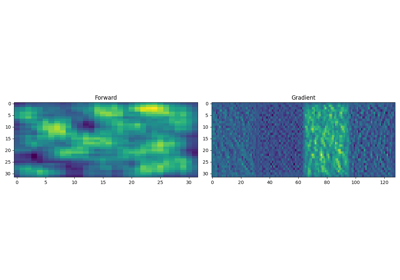# pylops.TorchOperator#

class pylops.TorchOperator(Op, batch=False, flatten=True, device='cpu', devicetorch='cpu')[source]#

Wrap a PyLops operator into a Torch function.

This class can be used to wrap a pylops operator into a torch function. Doing so, users can mix native torch functions (e.g. basic linear algebra operations, neural networks, etc.) and pylops operators.

Since all operators in PyLops are linear operators, a Torch function is simply implemented by using the forward operator for its forward pass and the adjoint operator for its backward (gradient) pass.

Parameters
Op`pylops.LinearOperator`

PyLops operator

batch`bool`, optional

Input has single sample (`False`) or batch of samples (`True`). If `batch==False` the input must be a 1-d Torch tensor or a tensor of size equal to `Op.dims`; if `batch==True` the input must be a 2-d Torch tensor with batches along the first dimension or a tensor of size equal to `[nbatch, *Op.dims]` where `nbatch` is the size of the batch

flatten`bool`, optional

Input is flattened along `Op.dims` (`True`) or not (`False`)

device`str`, optional

Device to be used when applying operator (`cpu` or `gpu`)

devicetorch`str`, optional

Device to be assigned the output of the operator to (any Torch-compatible device)

Methods

 `__init__`(Op[, batch, flatten, device, ...]) `apply`(x) Apply forward pass to input vector

## Examples using `pylops.TorchOperator`#19. Automatic Differentiation

19. Automatic Differentiation